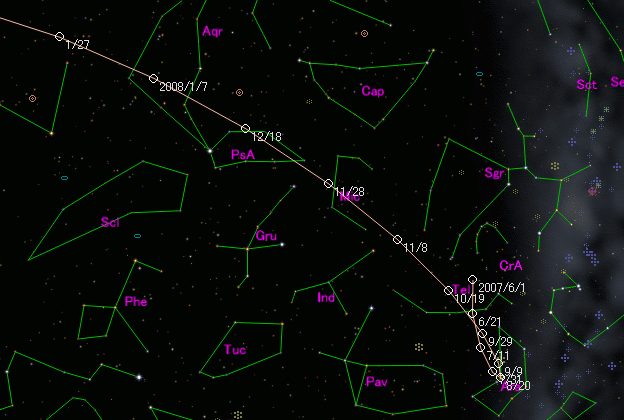# \$B%7%e!<%a!<%+!<(B-\$B%l%t%#Bh#1WB@1(B

192P/Shoemaker-Levy 1 (2007)###\$B%W%m%U%#!<%k(B

 \$BId9f(B 192P/2007 T3 \$B8!=PF|(B 2007\$BG/(B10\$B7n(B12\$BF|(B \$B8!=P8wEY(B 18.2\$BEy(B \$B8!=P R. H. McNaught (Siding Spring)

###\$B###\$B50F;MWAG(B

```                    Epoch = 2007 Dec.  6.0 TT
T = 2007 Dec. 17.3371 TT         Peri. = 312.8443
e = 0.773589                     Node  =  51.6501  2000.0
q = 1.460069 AU                  Incl. =  24.5612
a =  6.448748 AU    n = 0.0601854    P =  16.38 years
```

###\$B@1?^(B###\$B8wEYJQ2=(B

```        m1 = 9.0 + 5 log\$B&\$(B + 25 log r(t - 50)
```##### \$B50F;MWAG\$O!"(BIAUC 8879\$B\$K7G:\\$5\$l\$?\$b\$N\$G\$9!#(B \$B@1?^\$O%9%F%i%J%S%2!<%?(B Ver.8 (\$B%"%9%H%m%"!<%D(B) \$B\$G:n@.\$7\$?\$b\$N\$G\$9!#(B \$B8wEY%0%i%U\$O(BComet for Windows\$B\$G:n@.\$7\$?\$b\$N\$G\$9!#(B